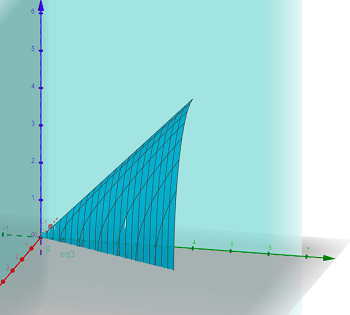# Sketch the solid on which the following integration is performed. Rewrite the integration using...

## Question:

Sketch the solid on which the following integration is performed. Rewrite the integration using the order dxdydz:

{eq}f^2_{0}\ f^4_{2x}\ f^\sqrt{y^2 - 4x^2 }_0\ dzdydz . {/eq}

## Iterated Integrals:

Recall that an iterated integral is one that contains a nest of integrals. There are a number of different ways that we can write an iterated integral, one for each order of integration. So for example, there are six different ways to write a triple integral.

The surface is given entirely by the upper bound on {eq}z {/eq}:

{eq}\begin{align*} z^2 &= y^2 - 4x^2 \end{align*} {/eq}

but that we are dealing only with the part of it that lies in the first octant. Note that we get the bounds on {eq}y {/eq} by first setting {eq}z = 0 {/eq} to get {eq}y = 2x {/eq}, then {eq}z = 0 {/eq} and {eq}x = 2 {/eq} to get {eq}y = 4 {/eq}.The volume is given by

{eq}\begin{align*} \int^2_{0}\int^4_{2x}\int^{\sqrt{y^2 - 4x^2 }}_0 dz\ dy\ dx \end{align*} {/eq}

to reverse the order, we write the cone as

{eq}\begin{align*} z^2 &= y^2 - 4x^2 \\ 4x^2 &= y^2 - z^2 \\ x &= \frac12 \sqrt{y^2 - z^2} \end{align*} {/eq}

Then looking at the {eq}yz {/eq}-plane, i.e. {eq}x = 0 {/eq}, we have

{eq}\begin{align*} z^2 &= y^2 \\ y &= z \end{align*} {/eq}

Then since {eq}y {/eq} is the upper bound before, we know that {eq}z = 4 {/eq} is the new one. Then the reversed integral is

{eq}\begin{align*} \int_0^4 \int_0^z \int_0^{\frac12 \sqrt{y^2-z^2}} dx\ dy\ dz \end{align*} {/eq}